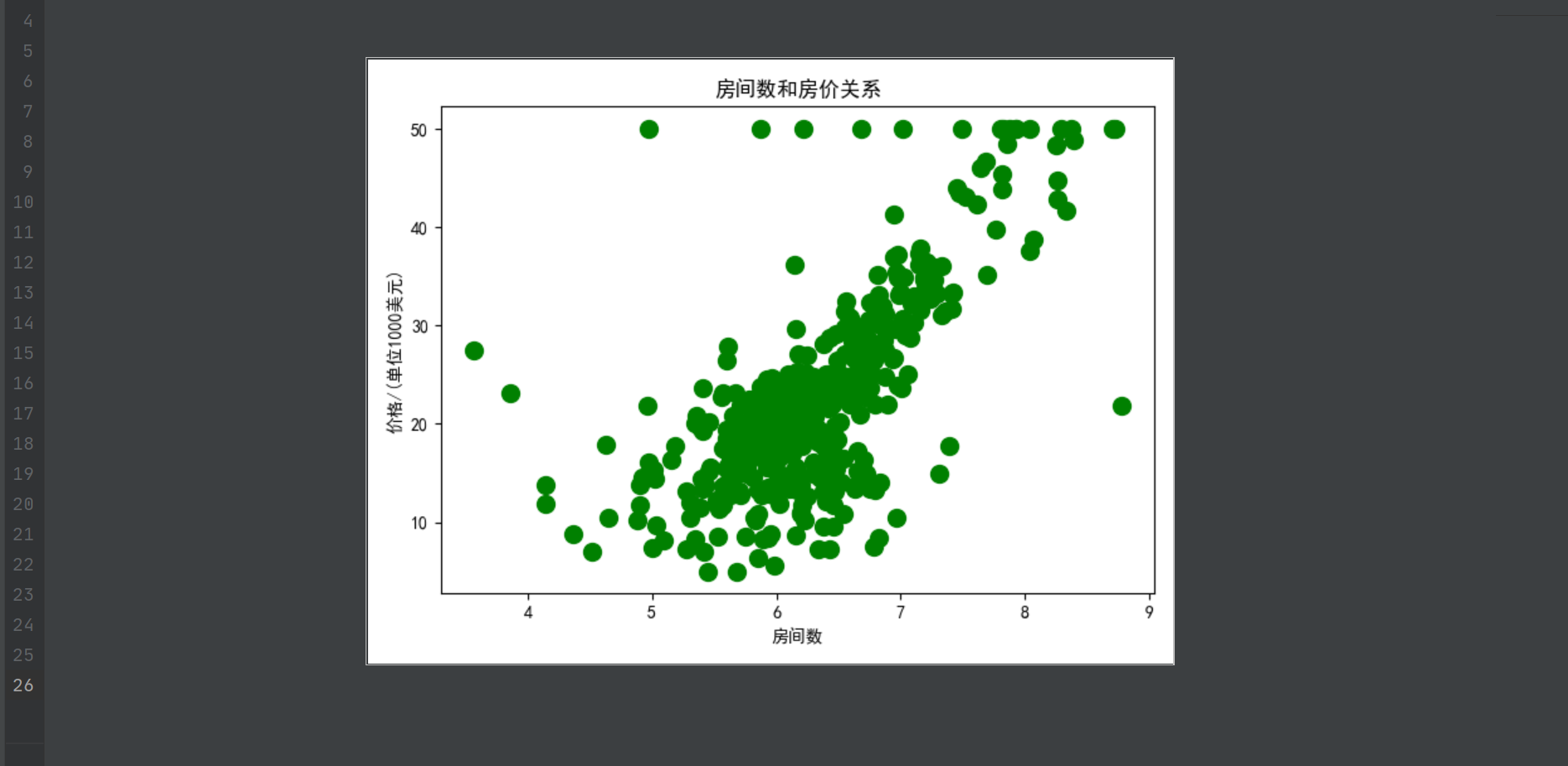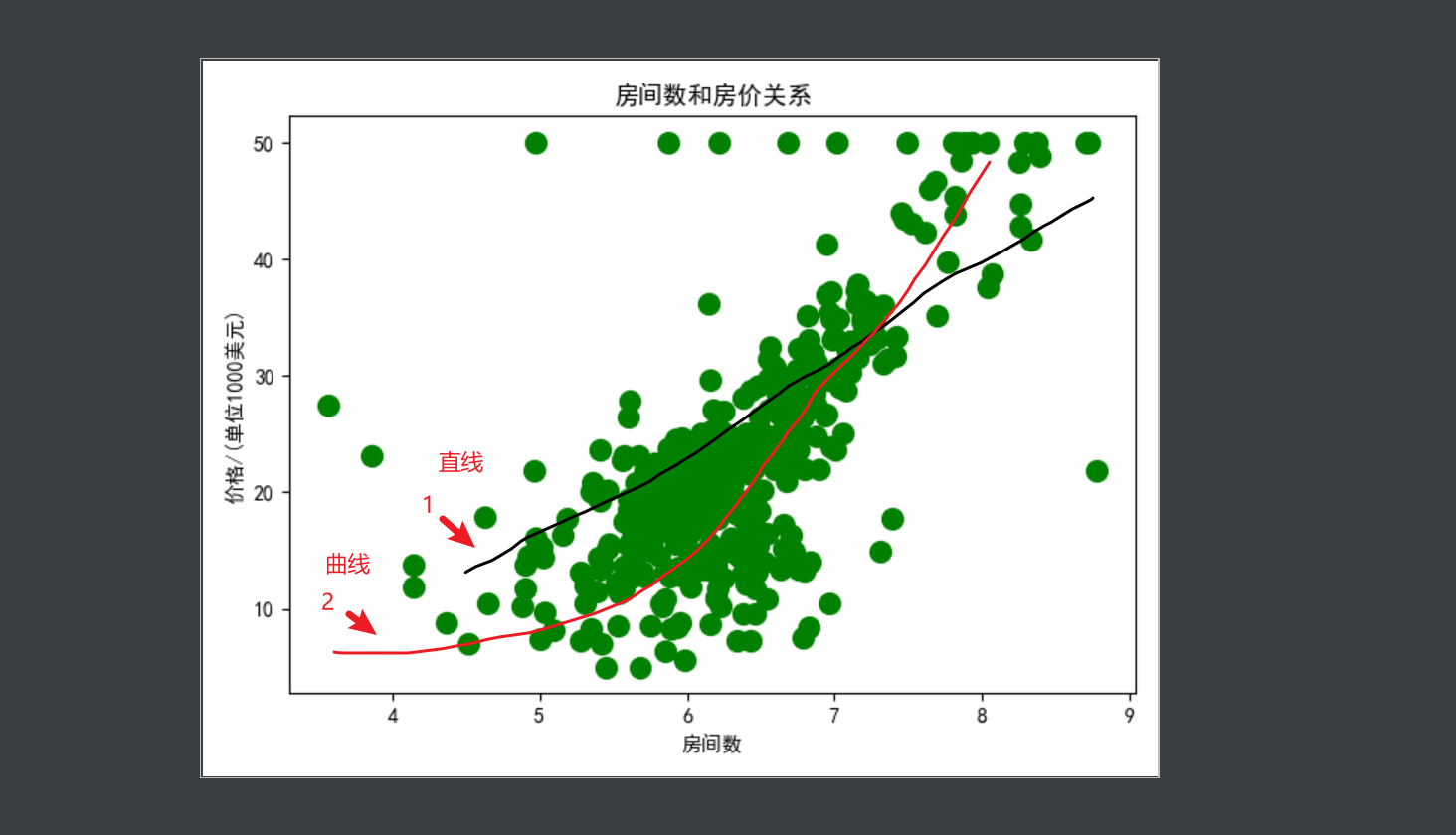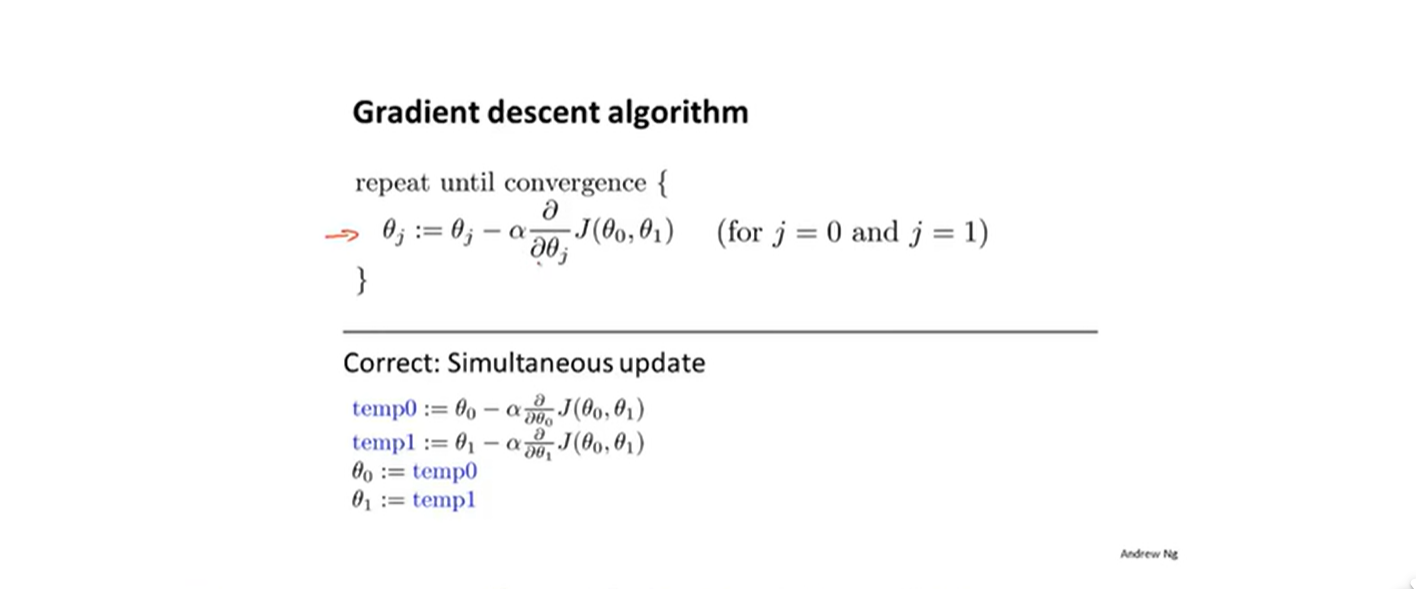### 机器学习梯度下降算法原理2022年8月14日 22:26ry222

import pandas as pd
import numpy as np
import matplotlib.pyplot as plt
plt.rcParams['font.sans-serif']=[u'SimHei']
plt.rcParams['axes.unicode_minus']=False

def notEmpty(s):
return s!=''
#格式
df = np.empty((len(data), 14))
for i, d in enumerate(data.values):
m = map(float, filter(notEmpty, d.split(' ')))
df[i] = list(m)
print(df.shape)
df1 = pd.DataFrame(df,columns=names)
room_num = df1['RM']
price_num = df1['MEDV']
print(room_num)
print(price_num)
plt.scatter(room_num,price_num,s=100,c='green')
plt.title('房间数和房价关系')
plt.xlabel('房间数')
plt.ylabel('价格/(单位1000美元)')
plt.show()，对于这样的3维图如何求J的极小值呢，这就得引入梯度的概念了，对于二维的函数，取极值直接进行求导即可(函数可导)，现在3维，可以分别对b0和b1方向分别求偏导，求得的偏导再矢量相加即为梯度，在数学意义上称为变化最快的方向了，那就很好理解了。梯度下降可以理解为每次往最快的方向移动，直到移动到最低点为止。对于代码我们可以分为2步，1给定初始值b0和b1，2不断同步更新b0和b1知道不变为止。